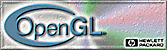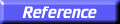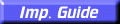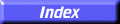# glMap1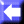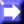glMap1d, glMap1f: define a one-dimensional evaluator.

C Specification | Parameters | Description | Notes | Errors | Associated Gets | See Also

##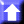C Specification

```void glMap1d(
GLenum	   target,
GLdouble	   u1,
GLdouble	   u2,
GLint	   stride,
GLint	   order,
const GLdouble *points)
void glMap1f(
GLenum	   target,
GLfloat	   u1,
GLfloat	   u2,
GLint	   stride,
GLint	   order,
const GLfloat  *points)
```

##Parameters

target
Specifies the kind of values that are generated by the evaluator. Symbolic constants GL_MAP1_VERTEX_3, GL_MAP1_VERTEX_4, GL_MAP1_INDEX, GL_MAP1_COLOR_4, GL_MAP1_NORMAL, GL_MAP1_TEXTURE_COORD_1, GL_MAP1_TEXTURE_COORD_2, GL_MAP1_TEXTURE_COORD_3, and GL_MAP1_TEXTURE_COORD_4 are accepted.
u1, u2
Specify a linear mapping of u, as presented to glEvalCoord1, to \$u hat\$, the variable that is evaluated by the equations specified by this command.
stride
Specifies the number of floats or doubles between the beginning of one control point and the beginning of the next one in the data structure referenced in points. This allows control points to be embedded in arbitrary data structures. The only constraint is that the values for a particular control point must occupy contiguous memory locations.
order
Specifies the number of control points. Must be positive.
points
Specifies a pointer to the array of control points.

##Description

Evaluators provide a way to use polynomial or rational polynomial mapping to produce vertices, normals, texture coordinates, and colors. The values produced by an evaluator are sent to further stages of GL processing just as if they had been presented using glVertex, glNormal, glTexCoord, and glColor commands, except that the generated values do not update the current normal, texture coordinates, or color.

All polynomial or rational polynomial splines of any degree (up to the maximum degree supported by the GL implementation) can be described using evaluators. These include almost all splines used in computer graphics: B-splines, Bezier curves, Hermite splines, and so on.

Evaluators define curves based on Bernstein polynomials. Define p(û ) as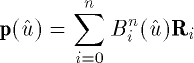where Ri is a control point and Bin(û ) is the ith Bernstein polynomial of degree n (order = n + 1):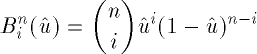Recall that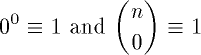glMap1 is used to define the basis and to specify what kind of values are produced. Once defined, a map can be enabled and disabled by calling glEnable and glDisable with the map name, one of the nine predefined values for target described below. glEvalCoord1 evaluates the one-dimensional maps that are enabled. When glEvalCoord1 presents a value u, the Bernstein functions are evaluated using û, where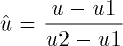target is a symbolic constant that indicates what kind of control points are provided in points, and what output is generated when the map is evaluated. It can assume one of nine predefined values:

GL_MAP1_VERTEX_3
Each control point is three floating-point values representing x, y, and z. Internal glVertex3 commands are generated when the map is evaluated.
GL_MAP1_VERTEX_4
Each control point is four floating-point values representing x, y, z, and w. Internal glVertex4 commands are generated when the map is evaluated.
GL_MAP1_INDEX
Each control point is a single floating-point value representing a color index. Internal glIndex commands are generated when the map is evaluated but the current index is not updated with the value of these glIndex commands.
GL_MAP1_COLOR_4
Each control point is four floating-point values representing red, green, blue, and alpha. Internal glColor4 commands are generated when the map is evaluated but the current color is not updated with the value of these glColor4 commands.
GL_MAP1_NORMAL
Each control point is three floating-point values representing the x, y, and z components of a normal vector. Internal glNormal commands are generated when the map is evaluated but the current normal is not updated with the value of these glNormal commands.
GL_MAP1_TEXTURE_COORD_1
Each control point is a single floating-point value representing the s texture coordinate. Internal glTexCoord1 commands are generated when the map is evaluated but the current texture coordinates are not updated with the value of these glTexCoord commands.
GL_MAP1_TEXTURE_COORD_2
Each control point is two floating-point values representing the s and t texture coordinates. Internal glTexCoord2 commands are generated when the map is evaluated but the current texture coordinates are not updated with the value of these glTexCoord commands.
GL_MAP1_TEXTURE_COORD_3
Each control point is three floating-point values representing the s, t, and r texture coordinates. Internal glTexCoord3 commands are generated when the map is evaluated but the current texture coordinates are not updated with the value of these glTexCoord commands.
GL_MAP1_TEXTURE_COORD_4
Each control point is four floating-point values representing the s, t, r, and q texture coordinates. Internal glTexCoord4 commands are generated when the map is evaluated but the current texture coordinates are not updated with the value of these glTexCoord commands.
stride, order, and points define the array addressing for accessing the control points. points is the location of the first control point, which occupies one, two, three, or four contiguous memory locations, depending on which map is being defined. order is the number of control points in the array. stride specifies how many float or double locations to advance the internal memory pointer to reach the next control point.

##Notes

As is the case with all GL commands that accept pointers to data, it is as if the contents of points were copied by glMap1 before glMap1 returns. Changes to the contents of points have no effect after glMap1 is called.

##Errors

• GL_INVALID_ENUM is generated if target is not an accepted value.
• GL_INVALID_VALUE is generated if u1 is equal to u2.
• GL_INVALID_VALUE is generated if stride is less than the number of values in a control point.
• GL_INVALID_VALUE is generated if order is less than 1 or greater than the return value of GL_MAX_EVAL_ORDER.
• GL_INVALID_OPERATION is generated if glMap1 is executed between the execution of glBegin and the corresponding execution of glEnd.

##Associated Gets

glGetMap
glGet with argument GL_MAX_EVAL_ORDER
glIsEnabled with argument GL_MAP1_VERTEX_3
glIsEnabled with argument GL_MAP1_VERTEX_4
glIsEnabled with argument GL_MAP1_INDEX
glIsEnabled with argument GL_MAP1_COLOR_4
glIsEnabled with argument GL_MAP1_NORMAL
glIsEnabled with argument GL_MAP1_TEXTURE_COORD_1
glIsEnabled with argument GL_MAP1_TEXTURE_COORD_2
glIsEnabled with argument GL_MAP1_TEXTURE_COORD_3
glIsEnabled with argument GL_MAP1_TEXTURE_COORD_4

glBegin,
glColor,
glEnable,
glEvalCoord,
glEvalMesh,
glEvalPoint,
glMap2,
glMapGrid,
glNormal,
glTexCoord,
glVertex